# 1490 and Level 2

### Today’s Puzzle:

You can solve this puzzle if you know the basic multiplication and division facts. Just write the numbers from 1 to 12 in both the first column and the top row so the given clues and those factors create a valid multiplication table.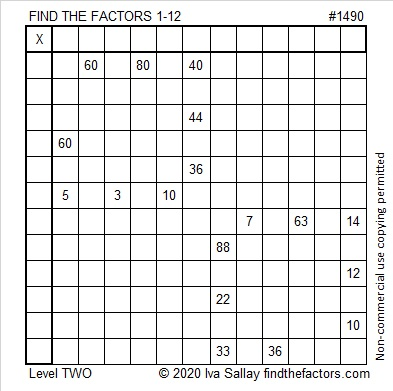### Factors of 1490:

• 1490 is a composite number.
• Prime factorization: 1490 = 2 × 5 × 149.
• 1490 has no exponents greater than 1 in its prime factorization, so √1490 cannot be simplified.
• The exponents in the prime factorization are 1, 1, and 1. Adding one to each exponent and multiplying we get (1 + 1)(1 + 1)(1 + 1) = 2 × 2 × 2 = 8. Therefore 1490 has exactly 8 factors.
• The factors of 1490 are outlined with their factor pair partners in the graphic below.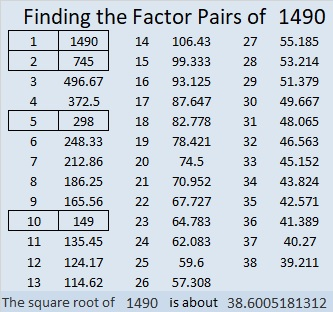### More Facts about the Number 1490:

1490 is the sum of two squares in two different ways:
31² + 23² = 1490.
37² + 11² = 1490.

1490 is the hypotenuse of FOUR Pythagorean triples:
432-1426-1490, calculated from 31² – 23², 2(31)(23), 31² + 23².
510-1400-1490, which is 10 times (51-140-149).
814-1248-1490, calculated from 2(37)(11), 37² – 11², 37² + 11².
894-1192-1490, which is (3-4-5) times 298.

# 1480 and Level 3

### Today’s Puzzle:

This is a level 3 puzzle. Start with the common factor of 21 and 14, then fill in the cells in the first column in order from top to bottom as well as the factors in the top row until you have placed all of the factors from 1 to 10 in both of those places.### Factors of 1480:

80 is divisible by 8 and 4 is even, so 1480 is divisible by 8. Here’s a little more about its factors:

• 1480 is a composite number.
• Prime factorization: 1480 = 2 × 2 × 2 × 5 × 37, which can be written 1480 = 2³ × 5 × 37
• 1480 has at least one exponent greater than 1 in its prime factorization so √1480 can be simplified. Taking the factor pair from the factor pair table below with the largest square number factor, we get √1480 = (√4)(√370) = 2√370
• The exponents in the prime factorization are 3,1 and 1. Adding one to each exponent and multiplying we get (3 + 1)(1 + 1) (1 + 1) = 4 × 2 × 2 = 16. Therefore 1480 has exactly 16 factors.
• The factors of 1480 are outlined with their factor pair partners in the graphic below.### Something else about the number 1480:

1480 is the hypotenuse of FOUR Pythagorean triples:
456-1408-1480 which is 8 times (57-176-185)
480-1400-1480 which is (12-35-37) times 40
832-1224-1480 which is 8 times (104-153-185)
888-1184-1480 which is (3-4-5) times 296

# 1473 A Level 5 Designed by My Granddaughter

### Today’s Puzzle:

My son and his family arrived today for a two-month long visit. We are so excited that they are here. His nine-year-old daughter has solved her share of Find the Factors puzzles. Today I gave her the opportunity to help me design one which she thoroughly loved doing. Here is the first of her creations. Will you be able to solve it? It’s a level 5 so you might find it a bit tricky.### Factors of 1473:

• 1473 is a composite number.
• Prime factorization: 1473 = 3 × 491
• 1473 has no exponents greater than 1 in its prime factorization, so √1473 cannot be simplified.
• The exponents in the prime factorization are 1, and 1. Adding one to each exponent and multiplying we get (1 + 1)(1 + 1) = 2 × 2 = 4. Therefore 1473 has exactly 4 factors.
• The factors of 1473 are outlined with their factor pair partners in the graphic below.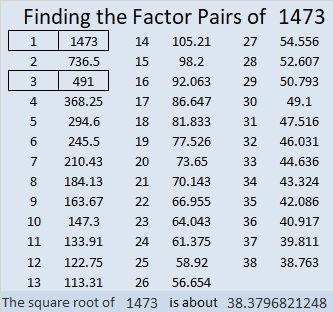### More about the number 1473:

1473 can be written as the difference of two squares in two different ways:
737² – 736² = 1473
247² – 244² = 1473

# 1472 and Level 4

### Today’s Puzzle:

Can you use logic to figure out where the factors from 1 to 12 must go to make the given clues be the products of those factors?### Factors of 1472:

• 1472 is a composite number.
• Prime factorization: 1472 = 2 × 2 × 2 × 2 × 2 × 2 × 23, which can be written 1472 = 2⁶ × 23.
• 1472 has at least one exponent greater than 1 in its prime factorization so √1472 can be simplified. Taking the factor pair from the factor pair table below with the largest square number factor, we get √1472 = (√64)(√23) = 8√23
• The exponents in the prime factorization are 6 and 1. Adding one to each exponent and multiplying we get (6 + 1)(1 + 1) = 7 × 2 = 14. Therefore 1472 has exactly 14 factors.
• The factors of 1472 are outlined with their factor pair partners in the graphic below.### A Little More about the Number 1472:

1472 is the difference of two squares in FIVE different ways:
369² – 367² = 1472
186² – 182² = 1472
96² – 88² = 1472
54² – 38² = 1472
39² – 7² = 1472

# 1471 and Level 3

### Today’s Puzzle:

There is only one factor that will work with the clue in the top row of this level 3 puzzle. Find it, then work your way down row by row until you have found all the factors. There is only one solution.### Factors of 147

• 1471 is a prime number.
• Prime factorization: 1471 is prime.
• 1471 has no exponents greater than 1 in its prime factorization, so √1471 cannot be simplified.
• The exponent in the prime factorization is 1. Adding one to that exponent we get (1 + 1) = 2. Therefore 1471 has exactly 2 factors.
• The factors of 1471 are outlined with their factor pair partners in the graphic below.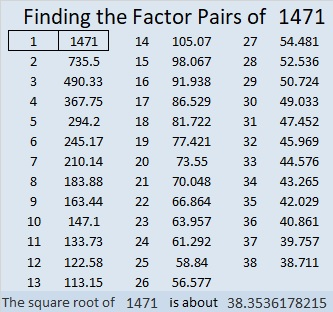How do we know that 1471 is a prime number? If 1471 were not a prime number, then it would be divisible by at least one prime number less than or equal to √1471. Since 1471 cannot be divided evenly by 2, 3, 5, 7, 11, 13, 17, 19, 23, 29, 31, or 37, we know that 1471 is a prime number.

### Another Fact about the Number 1471:

1471 is the 21st centered heptagonal number because
(7(21²)-7(21)+2)/2 = 1471.

# 1469 and Level 2

### Today’s Puzzle:

Where do the Factors 1-12 belong on this multiplication table puzzle?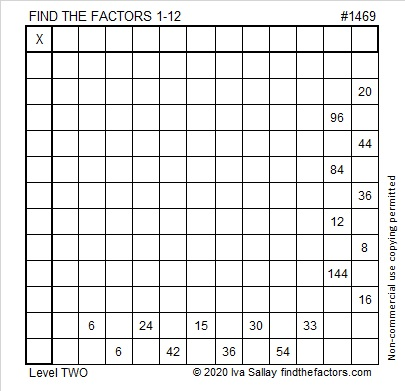### Factors of 1469:

• 1469 is a composite number.
• Prime factorization: 1469 = 13 × 113
• 1469 has no exponents greater than 1 in its prime factorization, so √1469 cannot be simplified.
• The exponents in the prime factorization are 1, and 1. Adding one to each exponent and multiplying we get (1 + 1)(1 + 1) = 2 × 2 = 4. Therefore 1469 has exactly 4 factors.
• The factors of 1469 are outlined with their factor pair partners in the graphic below.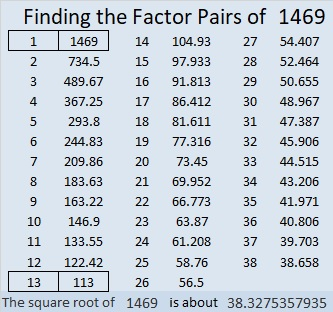### Other Facts about the Number 1469:

1469 is the sum of two squares in two different ways:
38² + 5² = 1469
37² + 10² = 1469

1469 is the hypotenuse of FOUR Pythagorean triples:
195-1456-1469 which is 13 times (15-112-113)
380-1419-1469 calculated from 2(38)(5), 38² – 5², 38² + 5²
565-1356-1469 which is (5-12-13) times 113
740-1269-1469 calculated from 2(37)(10), 37² – 10², 37² + 10²

# 1408 Powers of 2 in the Multiplication Table

number, puzzle, factors, factor pairs, prime factorization,

I have a 10 × 10 multiplication table poster in my classroom to help students who haven’t memorized the times table yet. We have to spend our time going over more advanced topics. One student struggled with the idea of raising two to a power. I went to the poster and boxed in all the powers of two on it. While I boxed them in, I recited, “2⁰ = 1, 2¹ = 2,
2² = 2×2 = 4,
2³ = 2×2×2 = 8,
2⁴ = 2×2×2×2= 16,
2⁵ = 2×2×2×2×2= 32,
2⁶ = 2×2×2×2×2×2=64.”

I liked the pattern those powers of two made on the poster so I made this 32×32 multiplication chart on my computer and continued the pattern.I expect the chart has many things for you to notice and wonder about. You could also do it with powers of 3, or another number, but you would need to use a much bigger multiplication table to show as many powers.

Now I’ll tell you a little bit about the number 1408.

1408 is not a power of 2, but it is 11 times a power of 2, specifically, it is 11 × 2⁷.

• 1408 is a composite number.
• Prime factorization: 1408 = 2 × 2 × 2 × 2 × 2 × 2 × 2 × 11, which can be written 1408 = 2⁷ × 11.
• 1408 has at least one exponent greater than 1 in its prime factorization so √1408 can be simplified. Taking the factor pair from the factor pair table below with the largest square number factor, we get √1408 = (√64)(√22) = 8√22.
• The exponents in the prime factorization are 7 and 1. Adding one to each exponent and multiplying we get (7 + 1)(1 + 1) = 8 × 2 = 16. Therefore 1408 has exactly 16 factors.
• The factors of 1408 are outlined with their factor pair partners in the graphic below.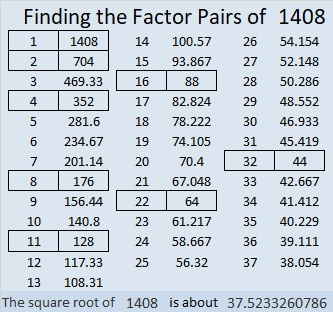Here is a festive multilayered factor cake for 1408: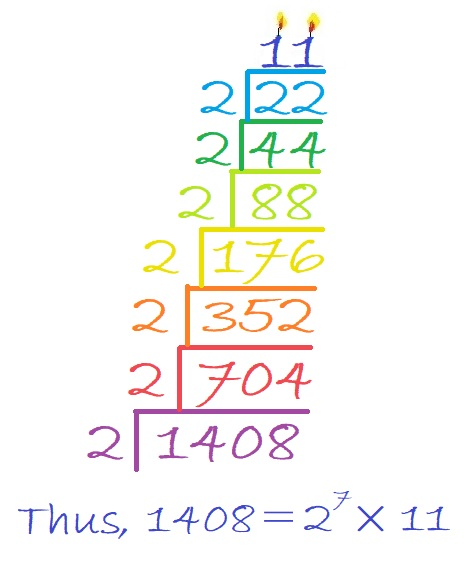So delicious! And here is a nicely balanced factor tree showing all of its prime factors: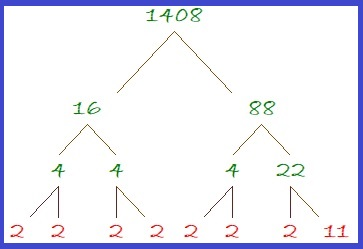# 1350 Logic is at the Heart of This Puzzle

### Today’s Puzzle:

By simply changing two clues of that recently published puzzle that I rejected, I was able to create a love-ly puzzle that can be solved entirely by logic. Can you figure out where to put the numbers from 1 to 12 in each of the four outlined areas that divide the puzzle into four equal sections? If you can, my heart might just skip a beat!

If you need some tips on how to get started on this puzzle, check out this video:

### Factors of 1350:

• 1350 is a composite number.
• Prime factorization: 1350 = 2 × 3 × 3 × 3 × 5 × 5, which can be written 1350 = 2 × 3³ × 5²
• The exponents in the prime factorization are 1, 3 and 2. Adding one to each and multiplying we get (1 + 1)(3 + 1)(2 + 1) = 2 × 4 × 3 = 24. Therefore 1350 has exactly 24 factors.
• Factors of 1350: 1, 2, 3, 5, 6, 9, 10, 15, 18, 25, 27, 30, 45, 50, 54, 75, 90, 135, 150, 225, 270, 450, 675, 1350
• Factor pairs: 1350 = 1 × 1350, 2 × 675, 3 × 450, 5 × 270, 6 × 225, 9 × 150, 10 × 135, 15 × 90, 18 × 75, 25 × 54, 27 × 50 or 30 × 45
• Taking the factor pair with the largest square number factor, we get √1350 = (√225)(√6) = 15√6 ≈ 36.74235

### Sum-Difference Puzzles:

6 has two factor pairs. One of those pairs adds up to 5, and the other one subtracts to 5. Put the factors in the appropriate boxes in the first puzzle.

1350 has twelve factor pairs. One of the factor pairs adds up to ­75, and a different one subtracts to 75. If you can identify those factor pairs, then you can solve the second puzzle!The second puzzle is really just the first puzzle in disguise. Why would I say that?

### More about the Number 1350:

1350 is the sum of consecutive prime numbers two ways:
It is the sum of the fourteen prime numbers from 67 to 131, and
673 + 677 = 1350

1350 is the hypotenuse of two Pythagorean triples:
810-1080-1350 which is (3-4-5) times 270
378-1296-1350 which is (7-24-25) times 54

1350 is also the 20th nonagonal number because 20(7 · 20 – 5)/2 = 1350

# 1226 Happy Birthday to My Sister, Sue

I don’t make puzzles bigger than 12 × 12 very often, but I decided to make this one, a 17 × 17 Mystery Level for my sister’s birthday. I know she can solve smaller ones without any problems, so I wanted to give her a challenge. Happy birthday, Sue. I hope you have a great day and enjoy solving this one.Print the puzzles or type the solution in this excel file: 10-factors-1221-1231

Note that with a bigger table there are several more possible common factors:

Is 4, 8, or 16 the common factor needed for 64 and 32 or for 16 and 48?
Is 7 or 14 the common factor needed for 14 and 70?
Is 6, 10, or 15 the common factor needed for 60 and 90?

As always there is only one solution. The table below will help anyone not familiar with some of the lesser known multiplication facts needed to solve the puzzle.Now I’ll share some information about the number 1226:

• 1226 is a composite number.
• Prime factorization: 1226 = 2 × 613
• The exponents in the prime factorization are 1 and 1. Adding one to each and multiplying we get (1 + 1)(1 + 1) = 2 × 2 = 4. Therefore 1226 has exactly 4 factors.
• Factors of 1226: 1, 2, 613, 1226
• Factor pairs: 1226 = 1 × 1226 or 2 × 613
• 1226 has no square factors that allow its square root to be simplified. √1226 ≈ 35.01428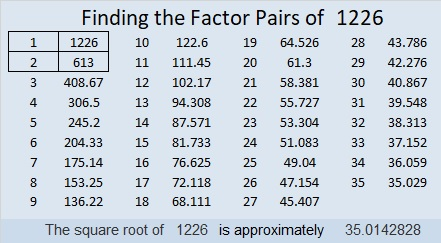35² + 1² = 1226

1226 is the hypotenuse of a Pythagorean triple:
70-1224-1226 calculated from 2(35)(1), 35² – 1², 35² + 1²

# 1161 and Level 1

Solving this puzzle will help you review the multiplication table. Knowing the multiplication table inside and out will be a big PLUS in your life. It will save you so much time in all your mathematics classes!Print the puzzles or type the solution in this excel file: 12 factors 1161-1173

Here is some information about the number 1161:

• 1161 is a composite number.
• Prime factorization: 1161 = 3 × 3 × 3 × 43, which can be written 1161 = 3³ × 43
• The exponents in the prime factorization are 3 and 1. Adding one to each and multiplying we get (3 + 1)(1 + 1) = 4 × 2 = 8. Therefore 1161 has exactly 8 factors.
• Factors of 1161: 1, 3, 9, 27, 43, 129, 387, 1161
• Factor pairs: 1161 = 1 × 1161, 3 × 387, 9 × 129, or 27 × 43
• Taking the factor pair with the largest square number factor, we get √1161 = (√9)(√129) = 3√129 ≈ 34.073451161 is the sum of the first twenty-six prime numbers. That’s all the primes from 2 to 101.

1161 is a palindrome in a couple of bases:
It’s 10010001001 in BASE 2 because 2¹⁰ + 2⁷ + 2³ + 2⁰ = 1161 and
1B1 in BASE 29 (B is 11 base 10) because 29² + 11(29) + 1 = 1161